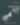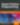# Creating CSS Animations Using Move.js

JavaScript

Share:

#### Free JavaScript Book!Write powerful, clean and maintainable JavaScript.

RRP \$11.95

CSS3 transitions and animations are currently the preferred method for creating lightweight animations on websites. Unfortunately, many developers find their syntax to be complex and confusing. If this sounds like you, Move.js may be the perfect solution for you. Move.js is a simple JavaScript library that creates CSS3 animations using simple functions. This tutorial explores the basics of Move.js, and provides a live demo of Move in action.

## The Basics

Move.js provides a simplified JavaScript API for creating CSS3 animations. Let’s assume that we have a `div` element of class `box`. We want to move it to 100px from the left when it is hovered over. In this case, our CSS code would look like this:

``````.box {
-webkit-transition: margin 1s;
-moz-transition: margin 1s;
-o-transition: margin 1s;
transition: margin 1s;
}
.box:hover {
margin-left: 100px;
}``````

With Move.js we can simply use the `set()` method to achieve the same results, as shown below.

``````move('.box')
.set('margin-left', 100)
.end();``````

## Getting Started

First, visit the Move.js GitHub page and download the latest package. Extract and copy the `move.js` file to your working directory. Next, include this file in your HTML page. A sample HTML file, with Move.js included, should look something like this:

``````<!DOCTYPE html>
<html>
<title>Move.js Demo</title>
<body>
<a href="#" id="playButton">Play</a>
<div class="box"></div>
<script type="text/javascript" src="js/move.js"></script>
</body>
</html>``````

We have defined a `div` element of class `box`, and a link with the ID `playButton` that will be used for our demo purpose. Let’s create a new `styles.css` file and add the following styles to it. Note that these styles are not needed by Move.js.

``````.box {
margin: 10px;
width: 100px;
height: 100px;
background: #7C9DD4;
}
#playButton {
display: block;
font-size: 20px;
margin: 20px 10px;
font-weight: bold;
color: #222;
text-decoration: none;
}``````

Our HTML page should now look like the following figure.Now, let’s write our first Move.js snippet. We need to attach an `onclick` handler to the Play button that will move the box 100px to the right when clicked. The JavaScript code for this hander is shown below. This code adds `transform: translateX(300px);` to the `box` element.

``````document.getElementById('playButton').onclick = function(e) {
move('.box')
.x(300)
.end();
};``````

The complete code, after adding the Move.js code, is shown below.

HTML

``````<!DOCTYPE html>
<html>
<title>Move.js Demo</title>
<body>
<a href="#" id="playButton">Play</a>
<div class="box"></div>
<script type="text/javascript" src="js/move.js"></script>
<script type="text/javascript">
document.getElementById('playButton').onclick = function(e){
move('.box')
.x(300)
.end();
};
</script>
</body>
</html>``````

CSS

``````.box {
margin-left: 10px;
width: 100px;
height: 100px;
background: #7C9DD4;
}
#playButton {
display: block;
font-size: 20px;
margin: 20px 10px;
font-weight: bold;
color: #222;
text-decoration: none;
}``````

## Move.js Methods

In the previous demo, we saw the `x()` method in action. Now, let’s learn about some of the other Move.js methods.

### `set(prop, val)`

The `set()` method is used to set CSS properties on the element in question. It takes two arguments, the CSS property and its value. Example usages would be:

``````.set('background-color', '#CCC')
.set('margin-left', 500)
.set('color', '#222')``````

### `add(prop, val)`

`add()` is used to increment a CSS property which is already set. The property must accept numeric values so that it can be incremented. This method takes two arguments, the CSS property and the amount of the increment.

``.add('margin-left', 200)``

When the previous snippet is called, it will add 200px to the existing value.

### `sub(prop, val)`

`sub()` is the inverse of `add()`. It accepts the same two arguments, however the value is subtracted from the property value.

``.sub('margin-left', 200)``

### `rotate(deg)`

As the name suggests, this method rotates the element by the amount provided as the argument. This methods attaches, the CSS `transform` property to the element when called. For example, the following code rotates an element by 90 degrees.

``.rotate(90)``

This code will add the following CSS to the HTML element.

``transform: rotate(90deg);``

### `duration(n)`

Using this method, you can specify how long the animation should take. For example, the following code tells Move.js to move the box to 200px from the left over two seconds.

``````.set('margin-left', 200)
.duration('2s')``````

Let’s look at another example. In the following code, Move.js will change the margin, set the background color, and rotate an element 90 degrees over two seconds.

``````.set('margin-left', 200)
.set('background-color', '#CCC')
.rotate(90)
.duration('2s')``````

### `translate(x[, y])`

`translate()` is used to change the position of an element from its default position, using the coordinates provided as the arguments. If only one argument is provided, it will be used as the x-coordinate. If a second argument is provided, it is used as the y-coordinate.

``.translate(200, 400)``

### `x()` and `y()`

Using `x()`, you can move adjust an element’s x-coordinate. Similarly, `y()` is used to move an element vertically. Both methods accept positive and negative values.

``````.x(300)
.y(-20)``````

### `skew(x, y)`

`skew()` turns an element to a provided angle with respect to both the x and y axis. This method can be segregated into `skewX(deg)` and `skewY(deg)`.

``.skew(30, 40)``

### `scale(x, y)`

This methods enlarges or compresses the HTML element’s size, as per the value provided to it. It uses the CSS3 transform’s `scale` method.

``.scale(3, 3)``

The above snippet will increase the height and width of the HTML element by three times.

### `ease(fn)`

If you have used CSS3 transitions, you know about the `ease` function that is provided to the `transition` property. It specifies the behaviour of the transition. Various `ease` functions are `in`, `out`, `in-out`, `snap`, `cubic-bezeir`, etc. These functions can be provided using Move’s `ease()` method. For example:

``````.ease('in').x(400)
.ease('cubic-bezier(0,1,1,0)').x(400)``````

### `end()`

This method is used at the end of Move.js snippet. It marks the end of the animation. Technically, this method triggers the animation to play. The `end()` method also accepts an optional callback function. An example is shown below.

``````move('.box')
.set('background-color', 'red')
.duration(1000)
.end(function() {
});``````

### `delay(n)`

As the name implies, this method is used to delay the animation by a specified amount of time.

``````move('.box')
.set('background-color', 'red')
.delay(1000)
.end();``````

### `then()`

This is one of the most important functions in Move.js. It will be used to divide the animation into two sets, which are executed in order. For example, the following animation contains two steps, which are divided by the call to `then()`.

``````move('.box')
.set('background-color', 'red')
.x(500)
.then()
.y(400)
.set('background-color', 'green')
.end();``````

## Creating Complex Animation using Move.js

In this tutorial, we have written many basic animations to look at the individual methods. Now, we’ll create a more complex animation easily using Move.js. This demo will clarify most of the concepts of the Move.js. We are going to create the animation depicted on this demo page. The Move.js code for this animation is shown below.

``````move('.square')
.to(500, 200)
.rotate(180)
.scale(.5)
.set('background-color', '#FF0551')
.set('border-color', 'black')
.duration('3s')
.skew(50, -10)
.then()
.set('opacity', 0)
.duration('0.3s')
.scale(0.1)
.pop()
.end();``````

## Conclusion

Hopefully, this tutorial has given you a clear understanding of what Move.js is, and how it can create CSS3 animations. Move.js can also help you organize all the animation code properly in a single place. Anytime you want to fix an animation, you know where it is!### Syed Fazle Rahman

Web Designer with over 6 years of experience, including user experience and front end development. Currently, CEO and Co-Founder of Hashnode, a network of software developers. Has published two books: Jump Start Bootstrap and Jump Start Foundation for SitePoint Premium.

#### New books out now!Learn how Git works, and how to use it to streamline your workflow!Google, Netflix and ILM are Python users. Maybe you should too?